# Function Calculator

Instructions: Use this function calculator to simplify, compute and graph any function, showing all the steps. Please type a valid function in the form box below.Enter the function you want to calculate, simplify (Ex: 2x + sin(x)-1/3 x, etc.)(Optional) Minimum x(Optional) Maximum x

## A Function Calculator

This calculator will allow you to compute, simplify and graph any valid function you provide, showing all the simplification steps. You need to provide a valid function to the calculator. It can be something already simplified like f(x) = 2x + 3, it could be something more complex that requires simplification, such as f(x) = (1/3+1/4)x + x^2 - sin(1/5+1/6) + 3/4'.

When a valid function is provided, then you can simply click on the "Calculate" button, and the process of simplification and graphing of the function will be shown to you.

Functions are the most important objects in Algebra and Calculus, and being able to correctly calculate and simplify expressions can make all the difference.## How to calculate function?

The idea of calculating a function is simply based on the definition of a function, where for a given value $$x$$ gets assigned one 'image' that is called $$f(x)$$.

In the graph below you can see how one value "x" on the x-axis gets assigned a point "f(x)" on the y-axis: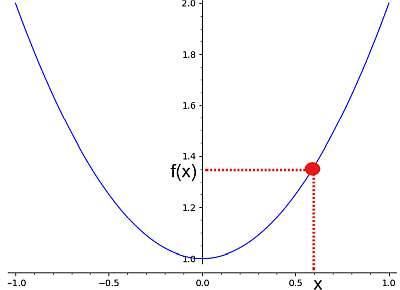So, the idea of a function calculation is to get a value "x" and be able to compute the value of "f(x)". Now, sometimes that is possible for some values of x, sometimes for all the x values in the real line. The set of values x where f(x) can be calculated is called the domain of a function.

## What are the steps for calculating a function?

• Step 1: Identify the expression that determines the function
• Step 2: Simplify the function as much as you can, but be aware of potential divisions by zero
• Step 3: Take note of where the function can and cannot be computed

So as you move along with this simplification process, you will have noted any values where the function cannot be evaluated (if any). This way, you have indirectly found the domain of the function.

For example, if you have a function such as f(x) = 2x + 1, regardless of the point you choose for x, the expression '2x + 1' can always be evaluated. But instead, if you have the function f(x) = 1/x, if you choose x = 0 you won't be able to compute the function at x = 0, because that would become 1/0, and a division by zero is undefined.

## How to simplify functions?

The process of simplification of function is just like any simplification of expressions: you use the criteria defined by the PEMDAS rule to conduct any potential simplification.

But there are a couple of caveats when using PEMDAS: you should avoid inadvertent divisions by zero, or taking square roots of negative numbers. For example, consider the function

$f(x) = \displaystyle\frac{2x}{x}$

You could think, well, I will cancel x, and then I get:

$\displaystyle f(x) = \displaystyle \frac{2\cancel{x}}{\cancel{x}} = 2$

But in doing so you would be making a mistake, because such cancellation of x cannot happen when x = 0. What you could do is to explicitly write

$\displaystyle f(x) = \displaystyle \frac{2\cancel{x}}{\cancel{x}} = 2$

for $$x \ne 0$$, and undefined for $$x = 0$$.

## What are the steps for simplifying?

• Step 1: Identify the provided function, and make sure it is a symbolically valid expression
• Step 2: Simplify terms as much as possible using the PEMDAS rule, taking care of not getting any divisions by zero, or negative square roots
• Step 3: Take note of those points where the function cannot be evaluated. The domain of the function will be the complement to those points in the real line

often times, it is pretty easy to spot points where there could be a problem at evaluating the function, by simple inspection of the structure of the function.

## Can you calculate a function from points?

It depends. The process of finding a function from given points is called interpolation. Now, for a given set of points, there will be more than one function that passes through those points, so in a way, giving points alone will not necessarily determine ONE function.

Now, adding certain constraints could make the determination unique. For example, for two given points, there is only one linear function (linear affine, to be more precise) that passes through them. Or given any three points, there is only one quadratic function that passes through them.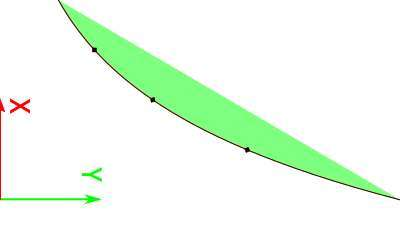### Example: Function calculation

Calculating and graph the function: $$f(x) = \frac{1}{3}x + \frac{5}{4}x^2 - \frac{5}{6}$$

Solution: The following function has been provided: $$\displaystyle f(x)=\frac{1}{3}x+\frac{5}{4}x^2-\frac{5}{6}$$, for which we need to simplify and construct its graph.

Step 0: In this case, we first need to simplify the given function $$\displaystyle f(x)=\frac{1}{3}x+\frac{5}{4}x^2-\frac{5}{6}$$, and in order to do so, we notice that:

$$\displaystyle \frac{1}{3}x+\frac{5}{4}x^2-\frac{5}{6}$$
Directly reorganizing/simplifying/expanding
$$= \,\,$$
$$\displaystyle \frac{5}{4}x^2+\frac{1}{3}x-\frac{5}{6}$$

The following plot is obtained for $$\displaystyle f(x)=\frac{5}{4}x^2+\frac{1}{3}x-\frac{5}{6}$$ on the interval $$[-5, 5]$$: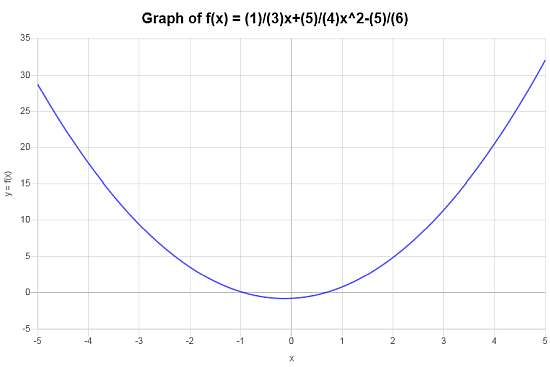### Example: Function calculator example

Calculate the domain of the following function : $$f(x) = \displaystyle \frac{x+1}{x^2-1}$$

Solution: The provided function $$\displaystyle f(x)=\frac{x+1}{x^2-1}$$ can be simplified as follows:

$f(x) = \displaystyle \frac{x+1}{x^2-1} = \displaystyle \frac{x+1}{(x-1)(x+1)} = \displaystyle \frac{1}{x-1}$

for when $$x \ne 1$$.Hence, the domain of the function is $$(-\infty, 1) \cup (1,\infty)$$. The following plot is obtained for the function on the interval $$[-5, 5]$$: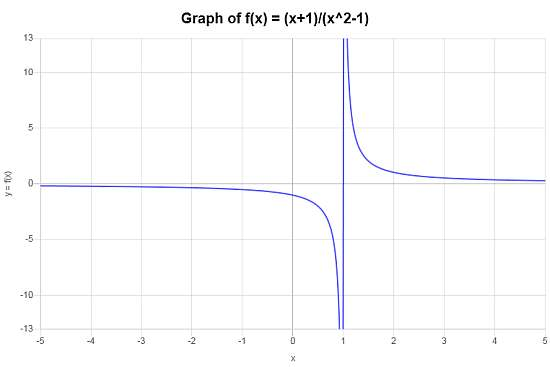### Example: Another function calculator example

Simplify and graph $$f(x) = \left(\frac{2}{3}x^2 \times \frac{6}{5} \right)+ e^{-x/10} + 2x^2$$.

Solution: We are provided with: $$\displaystyle f(x)=\frac{2}{3}x^2\cdot \frac{6}{5}+e^{\left(-1\right)x/10}+2x^2$$. Now, to simplify the given function $$\displaystyle f(x)=\frac{2}{3}x^2\cdot \frac{6}{5}+e^{\left(-1\right)x/10}+2x^2$$, we do:

$$\displaystyle \frac{2}{3}x^2\cdot \frac{6}{5}+e^{\left(-1\right)x/10}+2x^2$$
By expanding and simplifying the terms that allow simplification
$$= \,\,$$
$$\displaystyle \frac{14}{5}x^2+e^{\left(-1/10\right)x}$$

Therefore, following plot is obtained for $$\displaystyle f(x)=\frac{14}{5}x^2+e^{\left(-1/10\right)x}$$ on the interval $$[-5, 5]$$: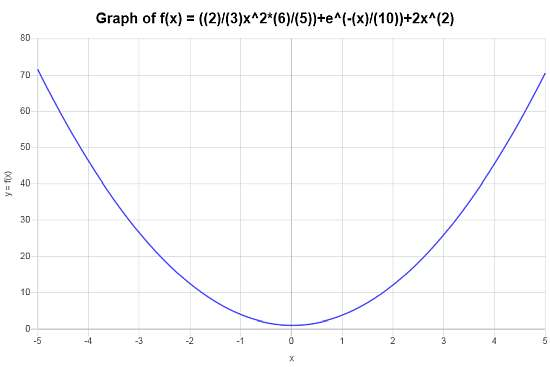## Other function calculators

The idea of function is central to Algebra and Calculus. There are plenty of things you can do with functions. One of the main abilities you can develop is to become comfortable simplifying expressions, so to reduce the given function into a simpler one.

Just make sure that you don't get trigger happy and end up cancelling zeros and taking square roots of negative numbers .

Also, you may want to just graph a function, so to get a better idea of how the function looks like and what its main properties are.